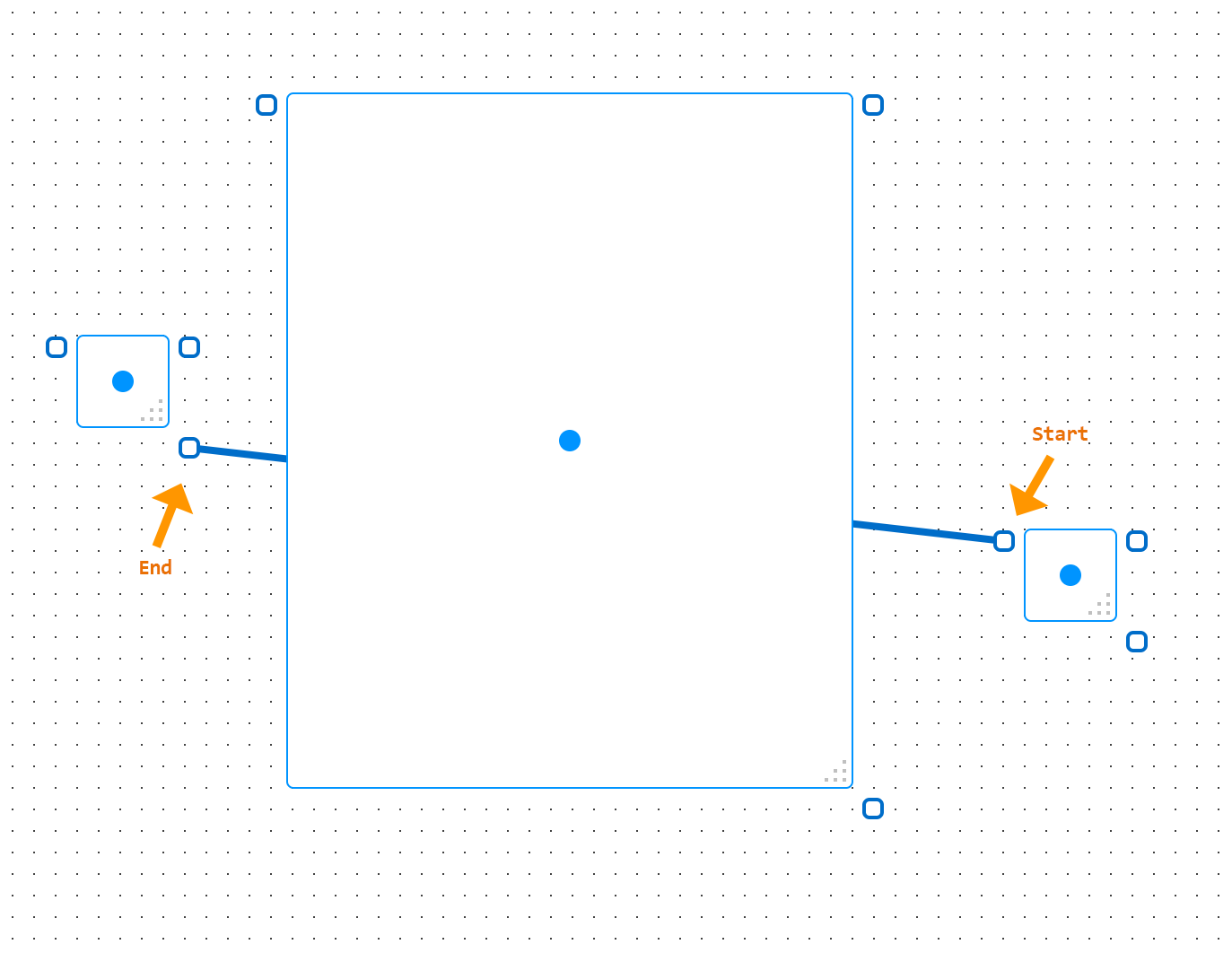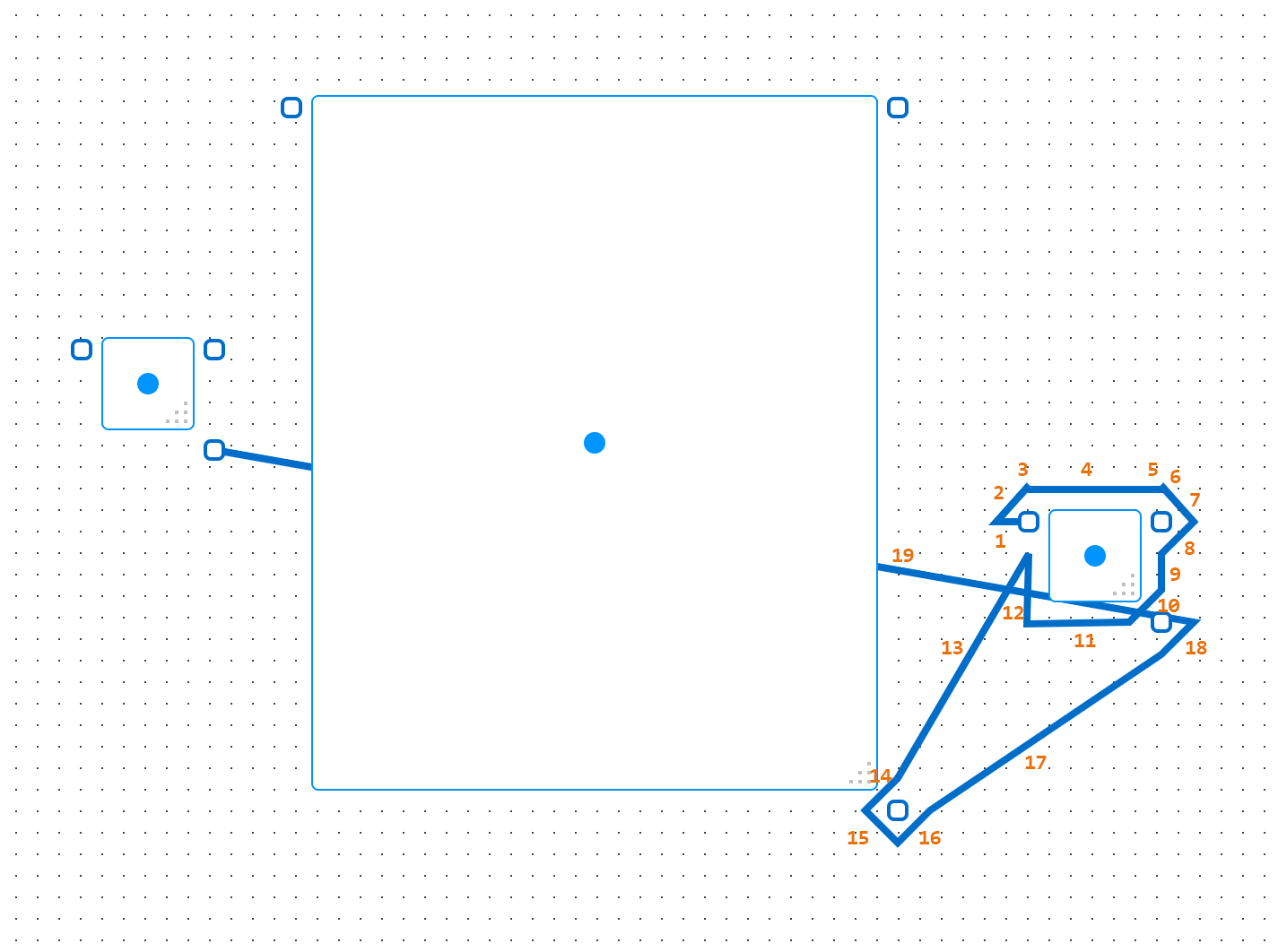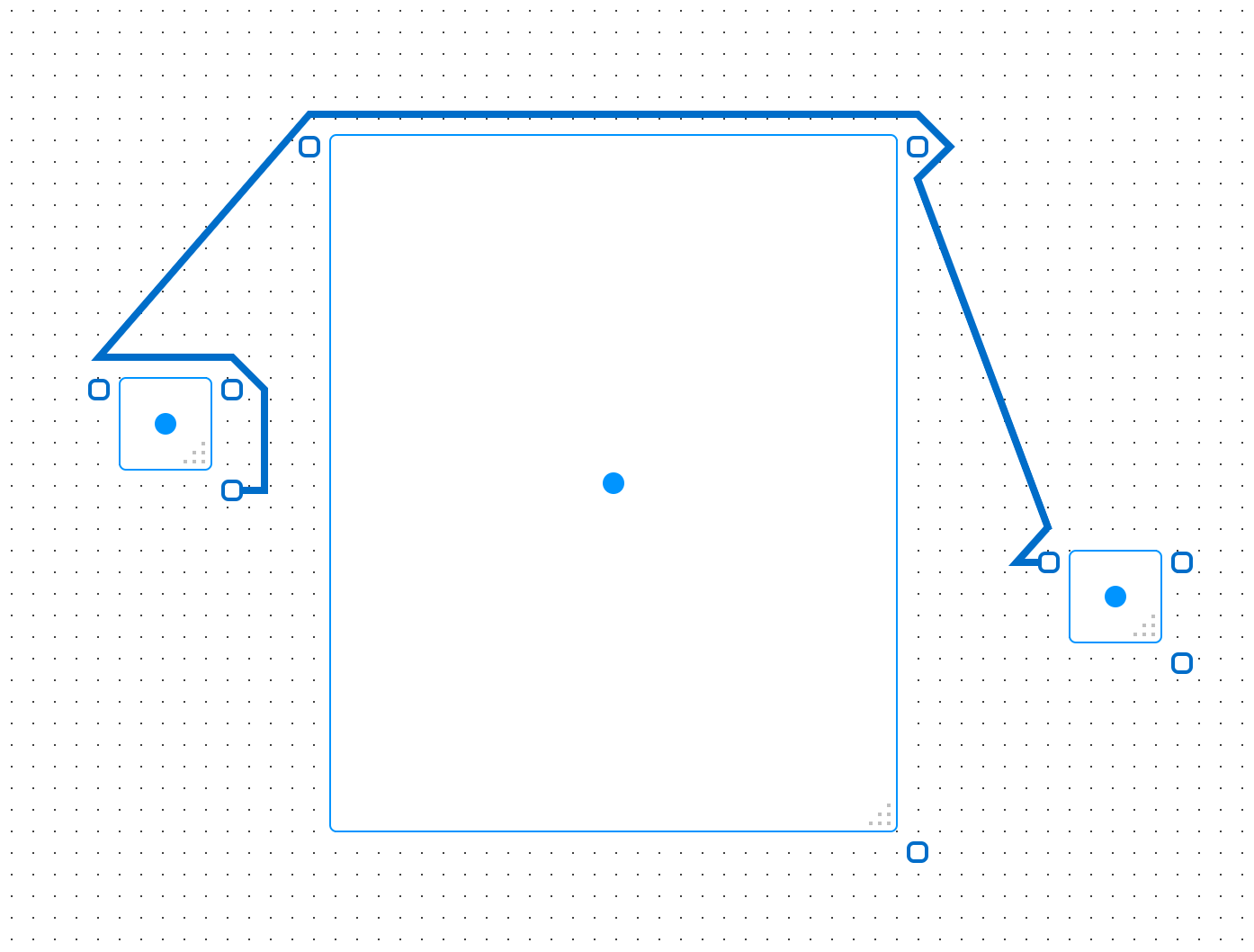## Archive for March, 2021

### Avoiding loops and routing failures with A*

#### The problem

With GraphPaper, generating path with the A* algorithm I ran into some interesting cases where the algorithm would fail to generate a path between the start and end points:Note that the path should go around, not directly across the big rectangle in the center.

There’s a few things being masked here, as GraphPaper will always try to produce a result, even if it’s non-optimal. So we need to disable:

• The mechanism that makes a path directly from start to end if there’s a failure in generating a path
• Path optimization

With these disabled, and some annotations showing the order in which the path segments were generated, we can get a better idea of what’s happening:Here we see that the algorithm generates a path that loops around its adjacent object (1-12), the path moves away from the object (13-14), then moves right back towards the object (15-18), then we’re out of accessible routing points, so we have a failure and we end the path by going directly to the endpoint. It’s important to note that routing points are not grid points here, they’re points designated around objects (the rectangles with the dots in the center) and anchors (the rectangles where paths start and end).

#### Why is this happening?

This might seem like a bug in the A* implementation but, as far as I can tell, the algorithm is performing as it should and the issue here comes down to the path cost computation done in the main loop. A* doesn’t actually define how to compute cost, but GraphPaper uses run-of-the-mill Euclidean distance as a metric.

In the A* main loop, the cost of going from our current point to a given point, n, is given by:g(n) is the total length of the path from the starting point to n (sum of the length of all pieces of the path). In practice, we keep track of the current path length, so we only need to compute and add the straight-line length from current to n:h(n) is the straight-line length from n to the goal:The issues we’re seeing surface as a result of f(n) being based solely on distance computations, note that:

• We may have a good point, n, it’s close to our goal but requires a large jump from our current location, the large increase in g(n) leads to another, closer, point being prioritized
• We may have a bad point, n, it’s further from our goal but only requires a small jump from our current location, h(n) increases a bit but the relatively small increase in g(n), leads to this point being prioritized over a better one

The implication here is also that shorter jumps become preferable, even if we end up going in the opposite direction of the goal, as such jumps are safer (i.e. less likely to lead to an blocked/invalid path).

#### Smarter routing

To improve the routing, I wanted to adjust the cost computation such that moving to points that and in the direction of (and thus closer) to the goal are prioritized, so I introduced a new term, t(n), in the cost computation:To compute t(n), we first compute the dot product of 2 normalized vectors:

• vecCurrentTo(endpt): from current to the endpoint, representing the ideal direction we should head in
• vecCurrentTo(n): from current to n, representing the direction to n

The dot product ranges from [-1, 1]; tending to -1 when n is in the opposite direction of the ideal, and tending to 1 when n is in the same direction as the ideal.

We then scale the dot product result as we can’t just bias our overall cost, f(n), by a range this small, so we scale by the straight-line length from current to n. Using this length as a scaling factor also serves to bias towards longer paths in good/positive directions directions.

In code, the functions g(n), h(n) and t(n) components are computed like this:

```// g(n) = length/cost of _startPoint to _vp + _currentRouteLength const currentToVisibleLength = (new Line(currentPoint, visiblePt)).getLength(); let gn = currentToVisibleLength + _currentRouteLength; // h(n) = length/cost of _vp to _endPoint let hn = (new Line(visiblePt, _endPoint)).getLength(); // t(n) = // a. get the relationship between the 2 vectors (dot product) // b. scale to give influence (scale by currentToVisibleLength) // .. using currentToVisibleLength as scaling factor is influence towards longer paths in good directions vs shorter paths in bad directions const vecToTargetIdeal = (new Vec2(_endPoint.getX() - currentPoint.getX(), _endPoint.getY() - currentPoint.getY())).normalize(); const vecVisibleToEndpt = (new Vec2(visiblePt.getX() - currentPoint.getX(), visiblePt.getY() - currentPoint.getY())).normalize(); const tn = vecToTargetIdeal.dot(vecVisibleToEndpt) * currentToVisibleLength;```

With the updated path cost computation, we successful get a path to the endpoint and, even with path optimization off, we get a much better path overall with less unnecessary points in the path and no looping around objects:It’s also noting how this performs as the position of the object changes (again, path optimization is disabled and the mechanism that makes a path directly from start to end on routing failure is also disabled):

Without t(n)

With t(n)

In pretty much every case, applying the t(n) factor to the path computation produces a better path with no failures in routing to the endpoint.